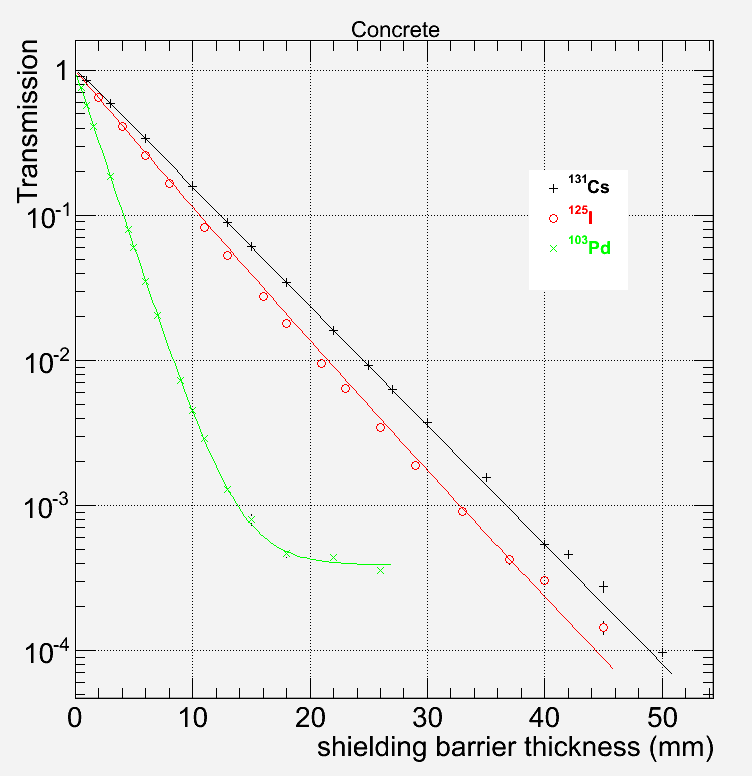CONCRETEMonte Carlo calculated radiation transmission data of this work for concrete shielding barrier of variable thickness, x. Presented lines correspond to results of fifting the three-parameter model of Eq. (1) in Ref. (1) to the data. See Introduction. Concrete barrier thickness required to achieve a given radiation transmission reduction, R, for Ir-192. Presented datasets correspond to calculations proposed in this work using the model parameters of Table II in Ref. (1), calculations using constant HVLe or TVLe value given in the IAEA Safety Report Series No. 47 (2006) (ref. 4), and calculations following the IAEA Report suggestion to use HVL1 or TVL1 up to R=102 and HVLe or TVLe thereafter. In the latter case, HVL and TVL values of this work, presented in Table III in ref (1), were used.